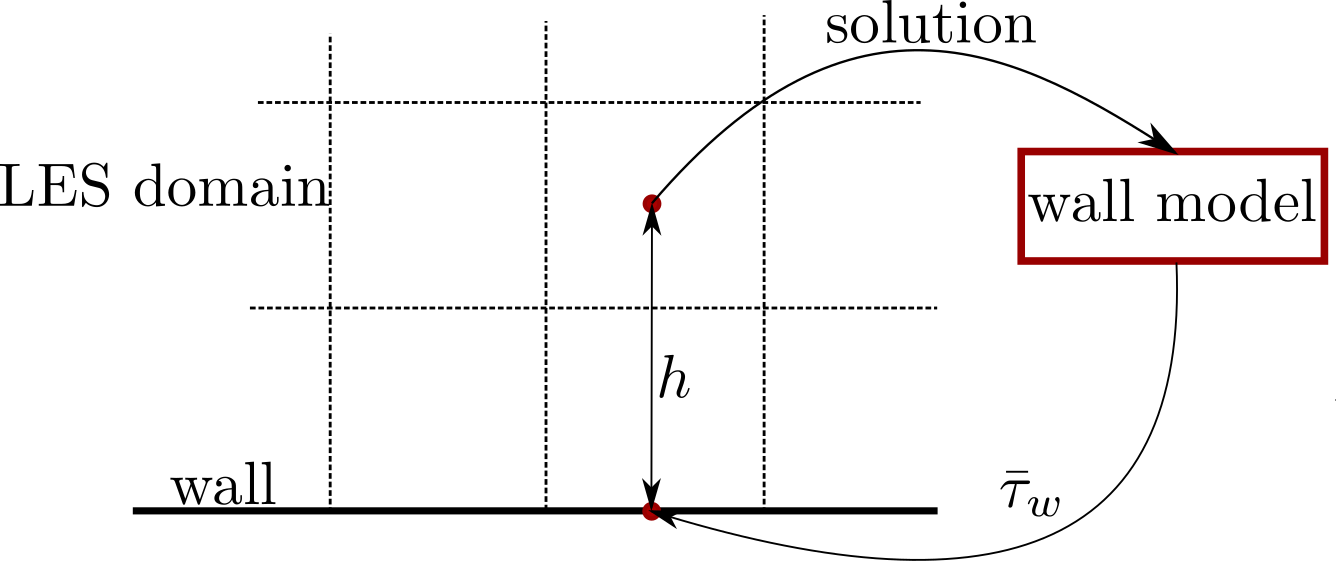# Wall modelling theory

## General considerations

Generally, WMLES implies modelling of the dynamics of the inner layer of turbulent boundary layers instead of resolving them with the grid. This entails significant computational savings at high Reynolds numbers, compared to performing a wall-resolved LES. A word of warning: WMLES is not cheap. Quite the opposite, WMLES is still expensive and is, for that reason, only starting to be used in applications, primarily in aerodynamical.

Note that the definition is rather general in the sense that it doesn’t define how the inner layer is to be modelled. On the other hand, it is quite specific since it applies only to attached boundary layers with a clear separation between outer and inner scales. This library only provides functionality for so-called wall stress modelling. This is a particular type of WMLES, in which the lack of inner layer resolution is compensated by providing the correct value of the wall shear stress. Providing this value is the task of the wall model. Wall stress modelling fits naturally into the numerical framework of OppenFOAM, i.e. finite volume discretization.

A schematic of how a wall-stress model works is shown in Fig. 1. For each wall face we consider some point away from the wall, located at a distance $$h$$. At this point, we can sample the solution to the LES equations, for example, the velocity and pressure gradient vectors. These sampled values are given to the wall model, which uses them to predict the wall shear stress $$\bar \tau_w$$.Fig. 1 Modus operandi of a wall-stress model.

This library provides associated functionality:

• Possibility to define an arbitrary $$h$$ for each face.

• Sampling relevant fields and temporal averaging of the sampled values.

• A set of wall models implemented as boundary conditions for nut.

Documentation on particular models is provided in the header .H files of corresponding classes. Below, a summary of the models is given and links to appropriate classes are provided.

## Algebraic models

These are essentially wall functions: some law of the wall is used to connect the sampled LES solution to the wall shear stress. Implemented in the library as the Foam::LOTWWallModelFvPatchScalarField class, see its documentation for further details. A multitude of laws of the wall are implemented:

The integrated versions are preferable if you use the wall-adjacent cell for sampling. Otherwise, there is no large difference in what law to use, and Spalding’s law is a reasonable default choice. The Newton root finder should be used to solve the associated non-linear algebraic equation.

## ODE-based models

These models are based on an ODE formulation, yet the ODE is integrated, and the model therefore only performs numerical integration using the trapezoidal rule, and does not solve and ODE directly. The models differ in the treatment of the right-hand side of the underlying ODE. For more details see the Foam::ODEWallModelFvPatchScalarField class.

The following models are available